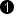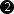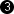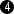## Operator CONST

The operator CONST provides a fixed value at the output link. All input values are discarded and replaced by the constant. The constant can be defined by parameter Value. This can either be a static or dynamic value. Thus the value can be changed during runtime.

Note that for multi component formats like RGB, the constant value is defined as a single value for the full pixel and not just the component.

Example: Output link RGB 24bit, constant value is set to 11862494 (0xB501DE). The output components will then be set to the following const values: R = 222 (0xDE), G = 01 (0x01) and B = 181 (0xB5). The constant concatenates the component values to a single value. The component 0 is placed in LSB area and the highest component in MSB area, i.e. for RGB, the constant value is {B-G-R}. Value = {component N-1, ..., component 0}, with N being the number of the components.

### I/O Properties

Property Value
Operator Type O

Bit Width [1, 64][1, 63]Arithmetic {unsigned, signed} {unsigned, signed}
Parallelism any as I
Kernel Columns any anyKernel Rows any anyImg Protocol {VALT_IMAGE2D, VALT_LINE1D, VALT_PIXEL0D, VALT_SIGNAL} as I
Color Format any any
Color Flavor any any
Max. Img Width any as I
Max. Img Height any as I
 The range of the input bit width is [1, 64]. For signed inputs, the range is [2, 64]. For unsigned color inputs, the range is [3, 63] and for signed color, the range is [6, 63]. The range of the output for unsigned arithmetic is [1, 63]. For signed outputs, the range is [2, 64]. For unsigned color outputs, the range is [3, 63] and for signed color, the range is [6, 63]. The same const value is used for all kernel components.

### Parameters

Value
Default 0
Range depends on arithmetic and bit width of the output link

This parameter defines the value at the output link. If the parameter is set to Static, the value is selected at design time. If the parameter is Dynamic, it is possible to alter the value during runtime.

### Examples of Use

The use of operator CONST is shown in the following examples:

• 'Laser Triangulation'

Examples - A high speed and robust laser line detection algorithm. The algorithm determines center of gravity coordinates to obtain sub-pixel resolution results.